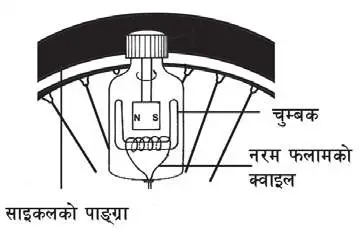# 3 and 4 Marks Question

1. Observe the given figure and answer the following questions.1. Which effect of current electricity is shown in the diagram?
2. Magnetic effect of current electricity is shown in the diagram.
3. What is this process called?
4. This process is called Electro magnetization.
5. What is the magnet so formed by this process called?
6. The magnet so formed by this process is called electromagnet.
7. How can we increase the strength of magnet formed by this method?
8. We increase the strength of magnet formed by this method by following ways:
1. By increasing the rotation of wire in coil.
2. By increasing the current in wire.
2. What type of transformer is given in the diagram? Why? Calculate the primary voltage of this transformer.3. Type of transformer is given in the diagram is step-up transformer because it has more number of turnings in secondary coil than primary coil.

Given,
V2 = 1100V
n2 = 500 turns
N1 = 100 turns
V1 = ?

We Know,
$\frac{\ce{V2}}{\ce{V1}}$ = $\frac{\ce{n2}}{\ce{n1}}$
or, $\ce{V1}$= $\frac{1100 × 100}{500}$
∴ V1 = 220V
4. In a house 6 bulbs each of 100 watt runs for 8 hours daily and 3 heaters of 1500 watt runs 5 hours daily, how much unit of electricity is consumed in thirty days?
5. Here,
For bulbs,
Electricity consumed (E1) = n × p × t
= $6 × \frac{100}{1000} × 8$
= 4.8 unit

For heater,
E2 = n × p × t
= $3 × \frac{1500}{1000} × 5$
= 22.5 unit

Total electricity consumed = E1 + E2
= 4.8 + 22.5
27.3 unit

Finally, electricity consumed in 30 days = 27.3 × 30
= 819 unit.

6. The number of turns in the primary winding of a certain transformer is 150 times more than that in the secondary winding. Calculate the input emf in the primary winding if the emf generated in the secondary winding is 220V AC. Which type of transformer is it? Why?
7. Here,
Let, the turnings in secondary coil (n1) = x turns
turnings in primary coil (n2) = 150x turns
Primary voltage (V1) = ?
Secondary voltage (V2) = 220V

We have, $\frac{\ce{V1}}{\ce{V2}}$ = $\frac{\ce{n1}}{\ce{n2}}$
or, $\frac{\ce{V1}}{220}$ = $\frac{150x}{x}$
∴ V1 = 3300V

This is step-down transfer because it has more number of turnings in primary coil than in secondary coil.
8. In a transformer the number of turns in primary coil is three times than the number of turns in secondary coil. If the primary voltage of transformer is 220 volts, find the secondary voltage.
9. Here,
Let, number of turns in secondary coil (n2) = x
number of turns in primary coil (n1) = 3x
Primary voltage (V1) = 220V
Secondary voltage (V2) = ?

We have,
$\frac{\ce{V2}}{\ce{V1}}$ = $\frac{\ce{n2}}{\ce{n1}}$

or, $\frac{\ce{V2}}{220}$ = $\frac{x}{3x}$
∴ V2 = 73.33V

10. In the given diagram C2 has 1/5 coil of C1. If the input voltage is 1200V, what will be the output voltage? What is the reason behind laminating the core of this device?11. Here,
V1 = 1200V
V2 = ?
n1 = n
n2 = n/5

Now, we know,
$\frac{\ce{V2}}{\ce{V1}}$ = $\frac{\ce{n2}}{\ce{n1}}$

or, $\ce{V2}$ = $\frac{\ce{n2 × V1}}{\ce{n1}}$

= $\frac{\ce{\frac{n}{5} × 1200}}{\ce{n}}$

∴V2 = 240V

Core of transformer is laminated with varnish and shellac so that it can prevent the flow of eddy current.

12. Answer the questions on the basis of given figure.1. Mention any two events that take place when the speed of bicycle wheel is increased.
2. Any two events that take place when the speed of bicycle wheel is increased are listed below:
1. Coil cuts the magnetic lines of force.
2. Current gets produced in the coil.
3. 'Working mechanism of this device is just opposite to the working mechanism of an electric motor.' Justify this statement.
4. Dynamo converts mechanical energy into electrical energy but the electric motor converts electrical energy into mechanical energy. Dynamo based on electromagnetic induction but electric motor based on motor effect. Hence, working mechanism of this device is just opposite to the working mechanism of an electric motor.
13. The number of turns in the secondary coil is 100 times more than that of primary coil. Calculate the output voltage, if 220 Volt input voltage is passed through this transformer. Where is this transformer used?
14. Here,
Let, the turnings in primary coil (n1) = x turns
turnings in secondary coil (n2) = 100x turns
Output voltage (V1) = 220V
Input voltage (V2) = ?

We have,
$\frac{\ce{V2}}{\ce{V1}}$ = $\frac{\ce{n2}}{\ce{n1}}$

or, $\frac{\ce{V2}}{220}$ = $\frac{100x}{x}$
or, V2 = 220 × 100
∴ V2 = 20000 Volt

This type of transfer is used in power station.

15. A transformer is shown in the figure.1. Label the parts A and B.
2. A = Primary Coil
B = Secondary Coil
3. Which type of transformer is it and why?
4. This is step-up transfer because it has more number of turnings in secondary coil than primary coil.
5. 'The use of alternating current (a.c.) is limited without transformer.' Justify it.
6. Voltage should be increased while distributing alternating current but it should be decreased while distributing at different home. Transformer is used for both purposes. Without transformer we cannot control the voltage for a.c. So, the use of alternating current (a.c.) is limited without transformer.